# closestPointsToSequence

Projects sequence of points onto path

Since R2022a

## Syntax

``pathPoints = closestPointsToSequence(refPath,points,initWindow)``
``[pathPoints,inWindow] = closestPointsToSequence(refPath,points,initWindow)``

## Description

````pathPoints = closestPointsToSequence(refPath,points,initWindow)` uses the closest point within a sequence of points, `points`, to be within the valid search window, `initWindow`. For each point in `Points`, the search window is centered at the previous point.```

example

````[pathPoints,inWindow] = closestPointsToSequence(refPath,points,initWindow)` optionally returns a logical vector `inWindow`, specifying whether each point for the corresponding xy coordinate in `points` is projected within the search window.```

## Examples

collapse all

`[refPath,generator,f0,f1] = closestPointExampleSetup;`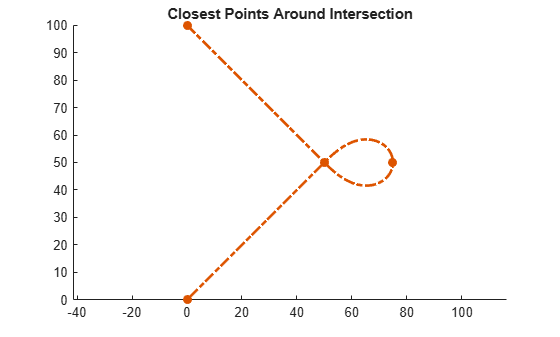Define the plot helper functions.

```mergeFcn = @(v1,v2)reshape([v1 v2 nan(size(v1,1),size(v2,2))]',[],1); plotFcn = @(L1,L2,linespec)plot(mergeFcn(L1(:,1),L2(:,1:min(1,size(L2,2)))), ... mergeFcn(L1(:,2),L2(:,2:min(2,size(L2,2)))),linespec{:}); plotInterval = @(bounds,nPt,linespec)plotFcn(interpolate(... refPath,linspace(bounds(1),bounds(2),nPt)'),[],linespec);```

Generate a trajectory which itself contains an intersection.

```f2 = f1; f2(1) = refPath.SegmentParameters(4,end) + 20;```

Set the states such that it starts from rest and terminates with a positive velocity and no acceleration.

```f0(2) = 0; % Initial lon. speed set to 0 f2(2) = 5; % Terminal lon. speed set to 5 deltaS = (f2(1)-f0(1)); % Longitudinal distance traveled vAvgEstimate = (f2(2)-f0(2))/2; % Rough average lon. velocity estimate T2 = deltaS/vAvgEstimate; % Ballpark travel duration```

Generate the trajectory.

```[fIntersecting,gIntersecting] = connect(generator,f0,f2,T2); gXingPts = gIntersecting.Trajectory;```

Plot the trajectory.

```figure show(refPath); xlim([0 125]) ylim([0 125]) hold on```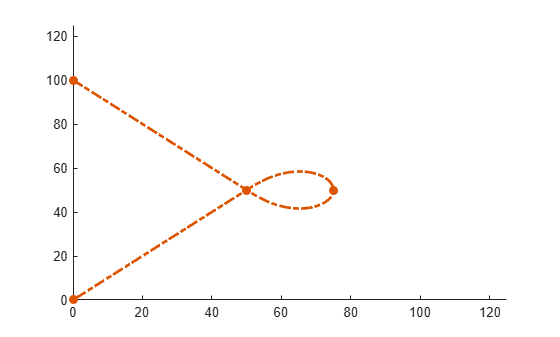Find the closest points across trajectory.

`closestPts = closestPoint(refPath,gXingPts);`

Find closest points in window that spans the full trajectory length.

```windowBuffer = 5; fXingPts = fIntersecting.Trajectory; fixedWindow = [min(fXingPts(:,1))-windowBuffer max(fXingPts(:,1))+windowBuffer]; closestPtsFullWindow = closestPoint(refPath,gXingPts,fixedWindow);```

Plot the naive closest-points here.

```title("Closest Points Along Self-Intersecting Trajectory") plotInterval(fixedWindow,100,{"Color",[.5 .5 .5],"LineWidth",5});```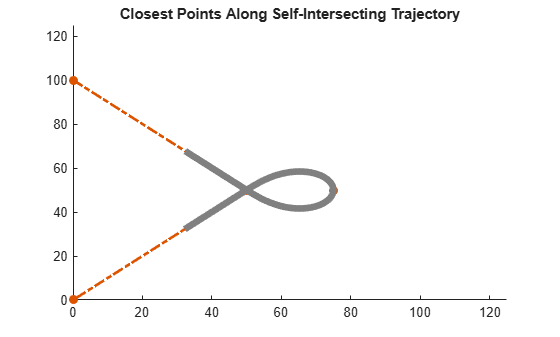Calculate a sliding window size based on the max global velocity. Note that this may not cover the trajectory depending on the shape of the reference path.

```dsApprox = max(abs(gXingPts(:,5)))*generator.TimeResolution; initialWindow = fXingPts(1)+[-1 1]*dsApprox; [closestPtsSlidingWindow, inWindow] = closestPointsToSequence(refPath,gXingPts,initialWindow);```

Calculate the region swept by the sliding window. Then plot the swept region.

```sweptRegion = [closestPtsSlidingWindow(1,end)-dsApprox,closestPtsSlidingWindow(end-1,end)+dsApprox]; plotInterval(sweptRegion,100,{":","Color",[.25 .25 .25],"LineWidth",3});```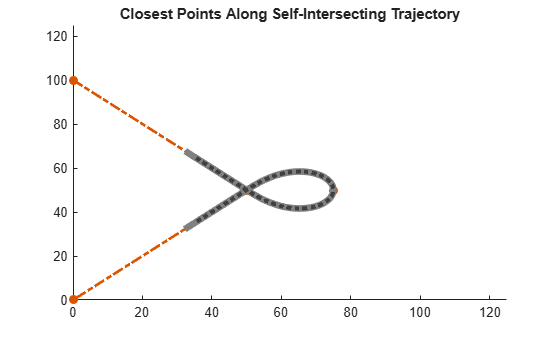```finalWindow = [closestPtsSlidingWindow(end-1,end)-dsApprox,closestPtsSlidingWindow(end-1,end)+dsApprox]; plotInterval(finalWindow,100,{"k","LineWidth",5});```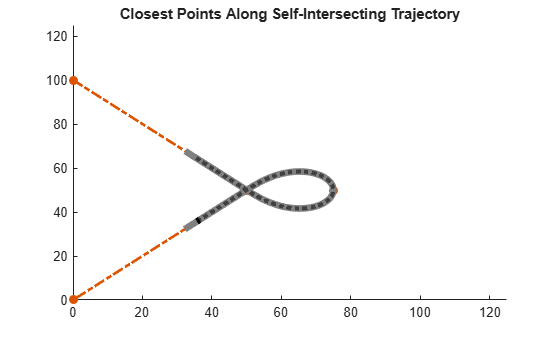Display results.

```plotFcn(gXingPts,[],{"k.-"}); plotFcn(gXingPts,closestPts,{"b","LineWidth",3}); plotFcn(gXingPts,closestPtsFullWindow,{"m","LineWidth",2}) plotFcn(gXingPts(inWindow,:),closestPtsSlidingWindow(inWindow,:),{"g"}); plotFcn(gXingPts(~inWindow,:),closestPtsSlidingWindow(~inWindow,:),{"r"}); legend({"Waypoints","ReferencePath","FixedWindow", ... "SlidingWindowSweptRegion","SlidingWindowFinalSpan", ... "Trajectory", "ClosestPoint","ClosestInsideFixedWindow", ... "ClosestInsideSlidingWindow"});```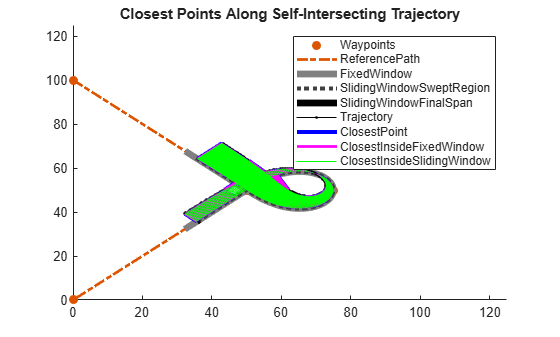## Input Arguments

collapse all

Reference path, specified as a `referencePathFrenet` object.

Global points, specified as a P-by-2 numeric matrix with rows of the form `[x y]`. P is the number of points. Positions are in meters.

Initial search window, specified as a two-element row vector in the form `[minimum_bound maximum_bound]` .

## Output Arguments

collapse all

Closest points on the reference path , returned as an N-by-6 numeric matrix with rows of form ```[x y theta kappa dkappa s]```, where:

• x y and theta — SE(2) state expressed in global coordinates, with x and y in meters and theta in radians

• kappa — Curvature, or inverse of the radius, in `m-1`

• dkappa — Derivative of curvature with respect to arc length in `m-2`

• s — Arc length, or distance along path from path origin, in meters

N is the number of points sampled along the reference path.

Indication whether each point nearest to the corresponding xy coordinate in `points`, is projected within the search window, returned as an N-element logical column vector, where N is the number of points in `points`. Points being projected within the search window are `true`, or `false` if they lie at the end of a window.

## Version History

Introduced in R2022a